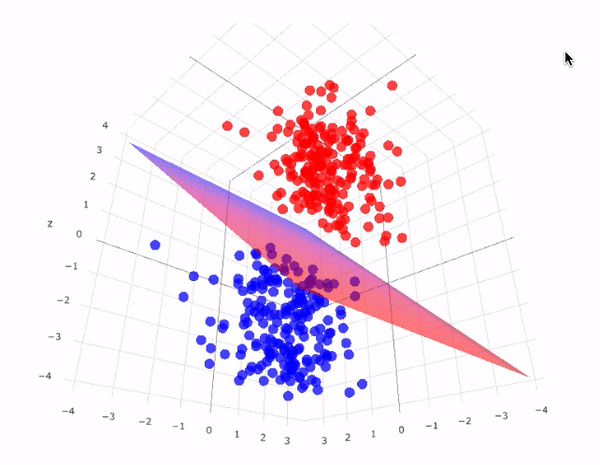# Support vector machine (SVM) algorithm in a fun & easy way

Original article was published on Artificial Intelligence on MediumIn your way to Learn machine learning, you must come across some fundamental machine learning algorithms such as linear regression, decision tree, and support vector machine, to be able to construct a solid understanding of the basics of the field, you could find the linear regression full introduction right here:

# What is Support Vector Machine?

SVM is a supervised machine learning algorithm that can be used for classification or regression problems. It uses a technique called the kernel trick to transform your data and then based on these transformations it finds an optimal boundary between the possible outputs. Simply put, it does some extremely complex data transformations, then figures out how to separate your data based on the labels or outputs you’ve defined.

How does SVM work?

The main objective is to segregate the given dataset in the best possible way. The distance between the nearest points is known as the margin. The objective is to select a hyperplane with the maximum possible margin between support vectors in the given dataset. SVM searches for the maximum marginal hyperplane in the following steps:

1. Generate hyperplanes that segregate the classes in the best way. Left-hand side figure showing three hyperplanes black, blue, and orange. Here, the blue and orange have higher classification errors, but the black is separating the two classes correctly.
2. Select the right hyperplane with the maximum segregation from either nearest data points

# Hyperplane

A hyperplane is a decision plane that separates between a set of objects having different class memberships.